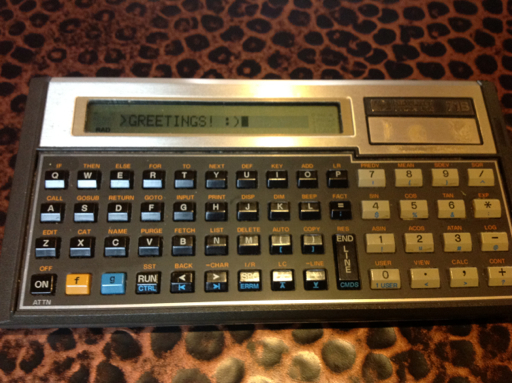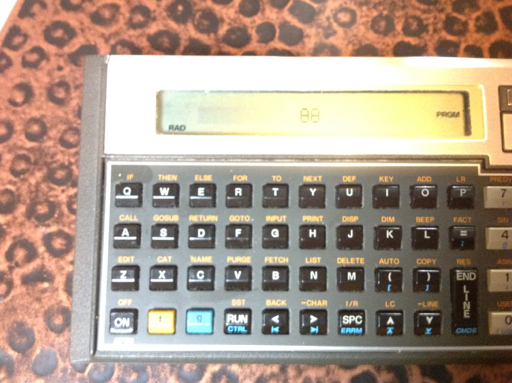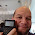## Sunday, June 3, 2012

### Fun With the HP 71B

This blog entry is dedicated to Namir Shammas, who just became a grandfather.

Greetings everyone! Recently I spent a week programming on an absolute beauty: the HP 71B calculator from Hewlett Packard. The calculator was manufactured during the mid 1980s, has a memory of over 16,000 bytes and four slots for software pacs or ROM PACs (cartridges). Currently I do not have any cartridges for the 71B at this time.

One cartridge gives the user access to the FORTH programming language on the 71B, which allows for the 71B to operate in RPN (Reverse Polish Notation) mode. Here is a video by AshLeaFen on 41 Translator ROM PAC on the 71B.

I won this calculator as a door prize, believe it or not, at a calculator convention last year.

BASIC PROGRAMMING

The primary feature of the HP 71B is its programming. The 71B has a QWERTY keyboard, which is just a pleasure to type on. The calculator's programming is versatile. To give a couple of examples:

Assigning a variable: I could use either a LET statement or just use the equals key by itself.

10 LET A = 1

or

10 A =1

The IF-THEN-ELSE structure. If there is just an integer after the THEN or ELSE, the calculator goes to that line without the need for a GOTO command. So:

50 IF X=1 THEN 100 ELSE 200

is just as effective as

50 IF X=1 THEN GOTO 100 ELSE 200

This trick works with line numbers only as I understand it.

I think preceding every line with line numbers is a lost art. While there may no longer be a need for them in today's languages, line numbers do give an order or structure to the program. The HP 33S and 35S programming calculators (also made by Hewlett Packard) keeps this tradition alive, except that line numbers are structured.

Below the picture of it are several of the programs I made over the last week. When it is not used, the 71B is stored in a Kindle case.LIST OF PROGRAMS

PROGRAM CTDWN (Countdown Timer)
11/16/2011 150 Bytes

10 DESTROY I
20 INPUT "# of seconds:";I
30 ON TIMER #1,1 GOSUB 60
40 DISP "Tick... Tock..."
50 IF I=1 THEN 90 ELSE 40
60 I=I-1
70 DISP I
80 RETURN
90 OFF TIMER #1 @ DISP I
100 WAIT I
110 DISP "Time's Up!"
120 END

This program uses the 71B's internal clock to make a countdown timer.

PROGRAM LIST1 (list of random integers)
158 bytes, 5/28/2012

10 OPTION BASE 1
20 DESTROY L
30 RANDOMIZE
40 INPUT "LENGTH? ";K
50 DIM L(K)
60 INPUT "HIGH NUMBER? "; N
70 FOR I=1 TO K
71 BEEP 512,.5
72 L(I)=IP(N*RND+1)
74 DISP "L(";I;")=";L(I)
76 WAIT 2
78 NEXT I
80 DISP "Done, check L."

The program generates a list of random integers from 1 to N. I have the 71B set the random seed to current time. Since this program leaves L as an array, if you want to use L as another variable, you probably have to execute DESTROY L first.

PROGRAM CARTH (Complex Number Arithmetic)
253 Bytes, 5/29/2012

10 DISP 'Complex Arithmetic'
20 INPUT "A,B(i),C,D(i):", A,B,C,D
30 DISP '1. + 2. - 3. * 4. /' @ WAIT 1.5
40 INPUT "Choice?"; H
50 ON H GOTO 60,70,80,90
60 DISP A+C;'+i';B+D @ END
70 DISP A-C;'+i';B-D @ END
80 DISP A*C-B*D;'+i';B*C+A*D @ END
90 DISP (A*C+B*D)/(C^2+D^2);'+i';(B*C-A*D)/(C^2+D^2) @ END

This is your basic arithmetic with complex numbers. The ON variable GOTO structure allows you to assign various GOTO commands in one line based on the value of variable.

PROGRAM RWALK (Random Walk of 50 Steps)
131 bytes; 5/30/12 - 50 steps - θ g CTRL P

10 DISP 'Random Walk'
15 DESTROY T,N,I
20 I=10 ! TAB POSITION
30 RANDOMIZE
40 FOR I=1 TO 50
42 N=IP(3*RND)-1
44 T=T+N
46 DISP TAB(T); 'θ'
48 NEXT I
50 WAIT 1
52 DISP 'Position:'; T

Where will the out of control θ end up?PROGRAM MATRIX1
238 Bytes, 11/16/2011

10 DESTROY A,B,C,D,E,A1,B1,C1,D1
20 INPUT "[[A,B][C,D]]:";A,B,C,D
30 E=A*D-B*C
40 DISP "DETERMINANT=";E
50 PAUSE
60 IF E=0 THEN STOP
70 A1=A/E @ B1=-B/E @ C1=-C/E @ D=D/E
80 DISP "INV="
90 WAIT 1
100 DISP "I11=";D1 @ WAIT 2
110 DISP "I12=";B1 @ WAIT 2
120 DISP "I21=";C1 @ WAIT 2
130 DISP "I22=";A1 @ WAIT 2
140 END

This program gives the determinant, and if it exists (det M ≠ 0), the inverse of a 2x2 matrix.

PROGRAM HORIZ (Horizontal Curve)
145 Bytes, 5/26/2012

100 DISP 'Horizontal Curves'
120 INPUT "Angle (DEG)? ";A
130 DEGREES
140 LET C=2*R*SIN(A/2)
150 DISP 'Chord Length=';C
155 PAUSE
170 DISP 'Chord Length=';L

A program for horizontal curves (engineering).

PROGRAM SUMFX (Σ f(x) a to b)
100+ bytes, 5/27/2012

Edit f(X) at line 10

10 DEF FNF(X)=insert function of X here
20 INPUT "Lower=";L
30 INPUT "Upper=";U
40 T=0
50 FOR X=L TO U @ T=T+FNF(X) @ NEXT X
60 DISP 'Total=';T

PROGRAM DERVX
100+ bytes, 5/27/2012

10 DEF FNF(X)=insert function of X here
20 INPUT "VALUE=";V
30 INPUT "TOLER 10^-";H
35 H=10^(-H)
40 D=(FNF(V+H)-FNF(V-H))/(2*H)
50 DISP "d/dX=";D

These programs calculate the sum of a function and the numeric derivative of a function, respectively. Line 10 is where you have to define your function (of X).

That concludes my blog entry for today.

P.S. Hewlett Packard, when will the HP 39gii calculator be on sale in the United States? Thanks!

This blog is property of Edward Shore. © 2012

1.Nice blog.How simple can you calculate cube root of a perfect cube using prime factorization method http://youtu.be/HeTMAPK6kf0

2.That is an interesting method.

3.Eddie thanks for all of your posts. I'm an HP enthusiast as well. I would like to chat. Could we swap contact info. Email rls43185@gmail or Facebook Jason Foose.

### Calculator Python: Lambda Functions

Calculator Python: Lambda Functions Introduction to Lambda Functions Lambda functions are a quick, one expression, one line, python function...